Skip To Content

# Analysis capabilities

See the following sections to learn which map or chart you should make to perform your analysis:

## Analyze your data

The tables in the sections below describe how you can do the following:

### Understand the quantities in your data

If you want to see the size, amount, or degree of a variable, use the following chart types:

IconChart typeRequirementsDescriptionHow to create it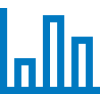Unique categories

Compare aggregated quantities between categories and identify broad differences at a glance.

Create a chart using a string field.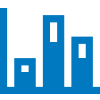Two unique categories

Compare aggregated quantities between categories or subcategories and identify broad differences at a glance.

Create a chart using two string fields.Treemap

Unique categories

Compare aggregated quantities in a hierarchical structure by comparing the size and placement of categories.

Create a chart using a string field.Bubble chart

Unique categories

Compare aggregated quantities across categories using bubble size to represent magnitude.

Create a chart using a string field.Heat chart

Two unique categories

Compare aggregated quantities as they intersect with two categories in a matrix form. Patterns in the data can be quickly discerned as the quantities change across cells.

Create a chart using two string fields.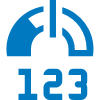Key Performance Indicator (KPI) card

Unique categories or numbers

Compare the measure of a key indicator to a target value.

Create a chart using a number, rate/ratio, or string field.

If you want to see the size, amount, or degree of data spatially, use the following map types:

IconMap typeRequirementsDescriptionHow to create itProportional symbol map

Numbers

Compare the quantities of your data within a spatial context. The size of the symbol corresponds to the magnitude of the data value.

Create a map using a number field.Aggregation map

Two spatial datasets

• One dataset with boundaries
• One dataset that will be aggregated into the boundaries

Compare aggregated quantities within a spatial context. A proportional symbol is assigned to each boundary area based on the magnitude of the aggregated data.

### Understand the relationships in your data

If you want to see the connection or similarity between variables, use the following chart types:

IconChart typeRequirementsDescriptionHow to create itChord diagram

Two unique categories

Visualize the relationships between categories and allow comparisons of similarities within a dataset or between different groups of data.

Create a chart using two string fields.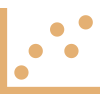Scatter plot

Two numbers

Assess the relationship between numbers visually and statistically, including the correlation between variables.

Create a chart using two number fields.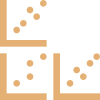Scatter plot matrix

Two or more numbers

Create multiple scatter plots at once to analyze the relationship between variables, while also comparing the relationships themselves.

Create a chart using three to five number fields.Link chart

Two unique categories

Visualize and assess the connections between nodes in a network.

Create a chart using two string fields.

If you want to see the connection or similarity of spatial data, use the following map types:

IconMap typeRequirementsDescriptionHow to create itChoropleth map

Proportions, rates, or ratios

Visualize the way ratios or proportions are distributed spatially to discern spatial patterns.

Create a map using a rate/ratio field, or using a number and normalizing the data.Flow map

Two location fields in the same dataset

Visualize and assess the magnitude and direction of connections between nodes in a network within a spatial context.

Create a link map with direction.Spider lines map

Either of the following:

• One dataset with two location fields
• Two datasets with location fields

Visualize and assess the magnitude of connections between nodes in a network within a spatial context.

Create a link map without direction, or use Find Nearest.

### Understand the change in your data

If you want to see how your data changes between categories or over time, use the following chart types:

IconChart typeRequirementsDescriptionHow to create itUnique categories

See the quantities of your categorical data and discern the differences between categories.

Create a chart using a string field.Two unique categories

See the quantities of your categorical data and discern the differences between and within categories.

Create a chart using two string fields.Heat chart

Two unique categories

Chart the intersection of categorical data to see trends in two dimensions.

Create a chart using two string fields.Data clock

Time data or categories

See the trends in your data over two different time intervals.

Create a chart using two date/time subfields.Line graph

Unique categories

See the values of your categorical data with an emphasis on trends between categories.

Create a chart using a string field.Time series graph

Time-enabled data

See how your data changes over time and discern patterns or trends.

Create a chart using a date/time field.Combo chart

Two datasets with the same unique categories

See the interaction between the quantities and trends in your data.

Create a line graph and a column chart on the same card.

If you want to see how your data changes spatially, use the following map types:

IconMap typeRequirementsDescriptionHow to create itProportional symbol map

Numbers

Visualize the quantities in your data and see the way those quantities change with location.

Create a map using a number field.Density map

Points

Calculate the density of your data and see how the density changes with location.Heat map

Points

Visualize spatial distributions based on areas with the most and least number of points, and how those distributions relate to location.

Create a map and change the Symbol type to Heat Map.Binned map

Points

Aggregate your data into equally sized bins to see overall spatial trends across your data.

Create a map and change the Symbol type to Bins.

### Understand the interactions in your data

If you want to see the flow of information between variables, use the following chart types:

IconChart typeRequirementsDescriptionHow to create itChord diagram

Two unique categories

See the quantity and direction of interactions between categories.

Create a chart using two string fields.Link chart

Two unique categories

Identify interactions and quantify the relationship of nodes relative to each other.

Create a chart using two string fields.

If you want to see the flow of information between places, use the following map types:

IconMap typeRequirementsDescriptionHow to create itFlow map

Two location fields in the same dataset

Identify relationships in spatial data and see the direction that information flows through a network.

Create a link map with direction.Spider lines map

Either of the following:

• One dataset with two location fields
• Two datasets with location fields

Identify relationships in spatial data, either through geographical proximity or attribute connections.

Create a link map without direction, or use Find Nearest.

### Understand the distribution of your data

If you want to see how your data is arranged numerically, use the following chart types:

IconChart typeRequirementsDescriptionHow to create it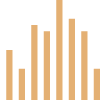Histogram

Numbers

See the distribution of your numerical data and make comparisons to a bell curve, or normal distribution.

Create a chart using a number field.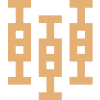Box plot

Numbers

See the distribution of your numerical data and identify basic statistical values.

Create a chart using a number field.

If you want to see how your data is arranged spatially, use the following map types:

IconMap typeRequirementsDescriptionHow to create itLocation map

Location field only

View your spatial data in its most basic form to identify where your data is located.

Create a map using a location field.Choropleth map

Proportions, rates, or ratios

Classify your proportional data to see how changes in the data interact with changes in location.

Create a map using a rate/ratio field, or using a number and normalizing the data.Proportional symbol map

Numbers

Classify your number data to see where the quantities are largest and smallest.

Create a map using a number field.Heat map

Points

Visualize your data based on the number of points and discern patterns based on spatial distribution.

Create a map and change Symbol type to Heat Map.Density map

Points

Calculate the density of your point data and see how the density is distributed spatially.Unique value map

Unique categories

See how your data is distributed and determine how categories are affected by location.

Create a map using a string field.Binned map

Points

Aggregate your data into equally sized bins to see a generalized view of how your data is distributed across locations.

Create a map and change Symbol type to Bins.

### Understand the proportions of your data

If you want to see the relative proportions of categories in your data, use the following chart types:

IconChart typeRequirementsDescriptionHow to create itDonut chart

Unique categories

See your categories arranged proportionally to see the size of each relative to the whole.

Create a chart using a string field.Treemap

Unique categories

See the proportions of your categories through both size and hierarchical orientation.

Create a chart using a string field.

## Make a map

If you have a spatial dataset, you're ready to make a map.

IconMap typeRequirementsDescriptionHow to create itLocation map

Location field only

Create a location map to see where your point, line, or area features are located.

Create a map using a location field.Aggregation map

Two spatial datasets

• One dataset with boundaries
• One dataset that will be aggregated into the boundaries

Create a spatial aggregation map to generalize your data and see big picture patterns.Binned map

Points

Create a binned map to see a quick, aggregated representation of how your data is distributed.

Create a map and change the Symbol type to Bins.Unique value map

Unique categories

Create a unique values map to see where point, line, or area features are located and what type they are.

Create a map using a string field.Choropleth map

Proportions, rates, or ratios

Create a choropleth map to see distributions of ratios or proportional data.

Create a map using a rate/ratio field, or using a number and normalizing the data.Density map

Points

Create a density map to determine the density distribution of your point data.Flow map

Two location fields in the same dataset

Create a flow map to see the magnitude and direction of relationships between different locations.

Create a link map with direction.Heat map

Points

Create a heat map to visualize areas with the most point features as the hottest.

Create a map and change the Symbol type to Heat Map.Proportional symbol map

Numbers

Create a proportional symbol map to show symbols of graduated sizes to indicate numeric values.

Create a map using a number field.Spider lines map

Either of the following:

• One dataset with two location fields
• Two datasets with location fields

Create a spider lines map to see the connections between different locations.

Create a link map without direction, or use Find Nearest.

## Make a chart

You can make a chart with any dataset, whether it's spatial or nonspatial.

IconChart typeRequirementsDescriptionHow to create itBox plot

Numbers

Create a box plot to see the distribution and basic statistics for your numerical data.

Create a chart using a number field.Bubble chart

Unique categories

Create a bubble chart to compare the quantities of your categorical data.

Create a chart using a string field.Unique categories

Create a bar or column chart to see an overview of your categorical data.

Create a chart using a string field.Combo chart

Two datasets with the same unique categories

Create a combo chart to see both quantities and trends for the same categorical variable.

Create a line graph and a column chart on the same card.Chord diagram

Two unique categories

Create a chord diagram to see directed relationships between categories.

Create a chart using two string fields.Data clock

Time data or categories

Create a data clock to see trends in your data over different periods of time.

Create a chart using two date/time subfields.Donut chart

Unique categories

Create a donut chart to see the proportions of your categorical data.

Create a chart using a string field.Heat chart

Two unique categories

Create a heat chart to see trends in the intersection of your categorical data.

Create a chart using two string fields.Histogram

Numbers

Create a histogram to see the frequency and distribution of your numeric data.

Create a chart using a number field.Key Performance Indicator (KPI) card

Unique categories or numbers

Compare the measure of a key indicator to a target value.

Create a chart using a number, rate/ratio, or string field.Line graph

Unique categories

Create a line graph to see trends between categories in your data.

Create a chart using a string field.Link chart

Two unique categories

Create a link chart to identify the interactions and relationships with your data.

Create a chart using two string fields.Scatter plot

Two numbers

Create a scatter plot to analyze the relationship and correlation between two numeric variables.

Create a chart using two number fields.Scatter plot matrix

Two or more numbers

Create a scatter plot to analyze the relationship and correlation between multiple numeric variables.

Create a chart using three to five number fields.Two unique categories

Create a bar or column chart to see an overview of your categorical data, including subgroups.

Create a chart using two string fields.Time series graph

Time-enabled data

Create a time series graph to see trends in your data over time.

Create a chart using a rate/ratio field.Treemap

Unique categories

Create a treemap to see the proportions of your categorical data through both size and hierarchical orientation.

Create a chart using a string field.